# 9 helpful tips and formulas in EXCEL

Jan 12, 2020 • edited Jan 13, 2020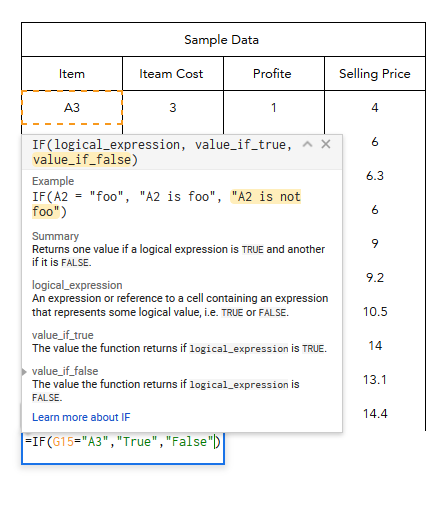1. Sum, Count, Average

=SUM(A1:A10)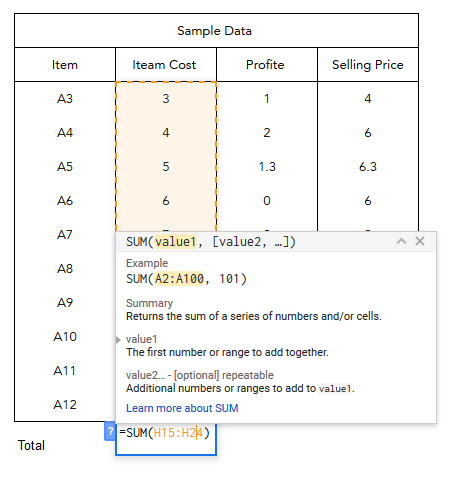Count

=Count(A1:A10)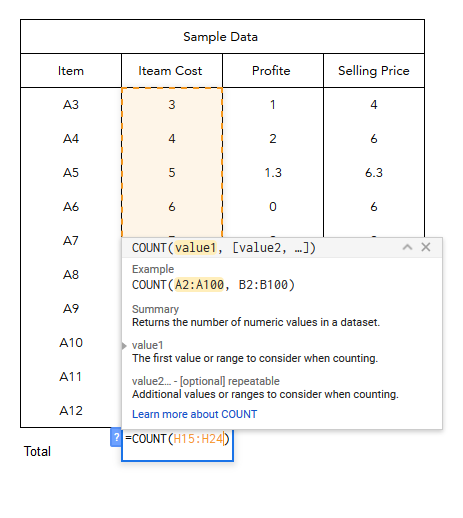Average

=Average( Value1, Value2)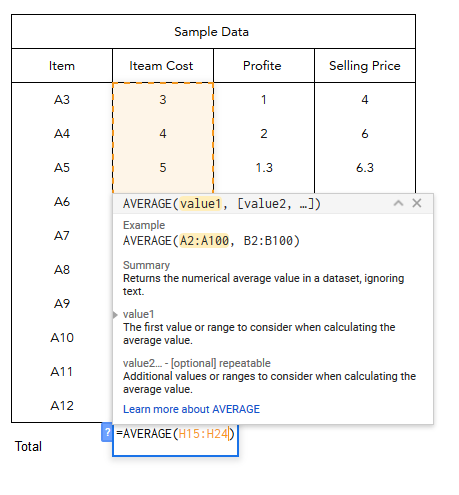1. If statements1. Sumif, Countif, Averageif

=SUM(A1:A15, “GOOD”, B1:B13)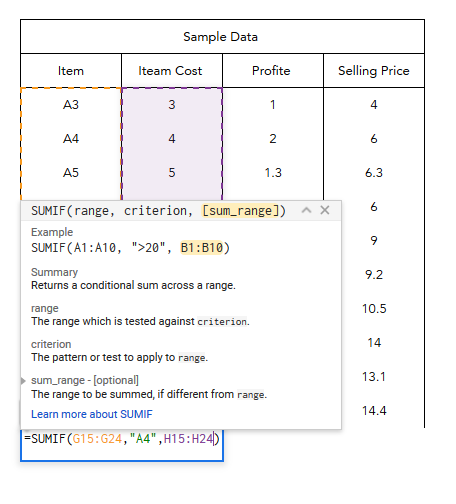=Countif(Range, Criteria, Function range)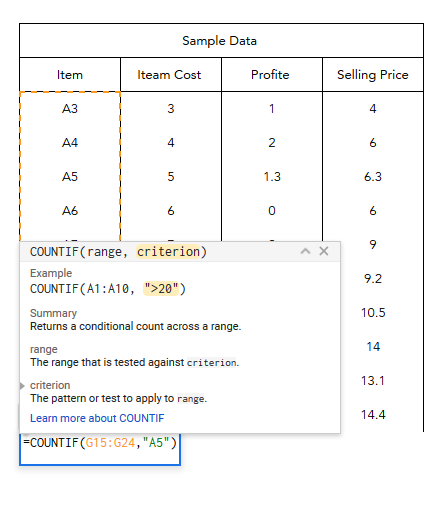=averageif( Range, Criteria, Function range )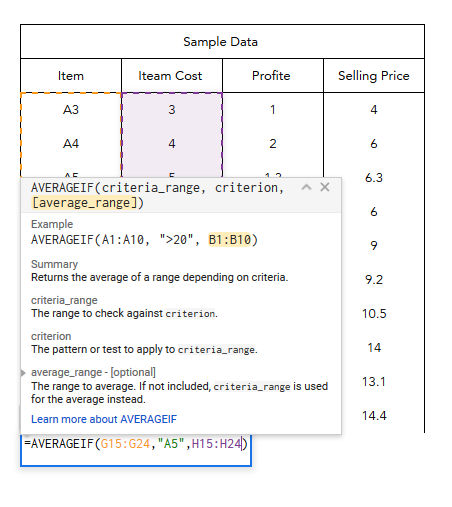1. VLookup

=VLOOKUP(lookup value, the table being searched, index number, sorting identifier)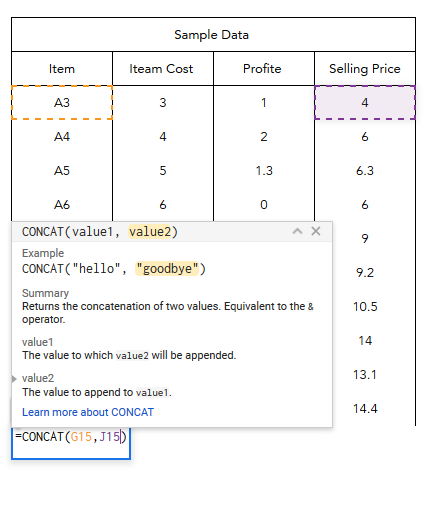1. Concatenate

=CONCATENATE(A1,” “,B2)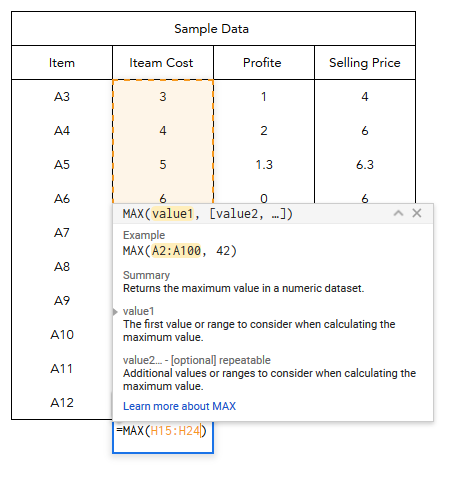1. Max and Min

=MAX(A1:A10)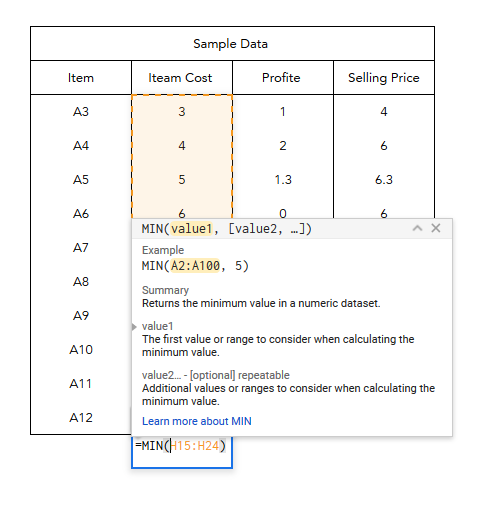Min =MIN(A1:A10)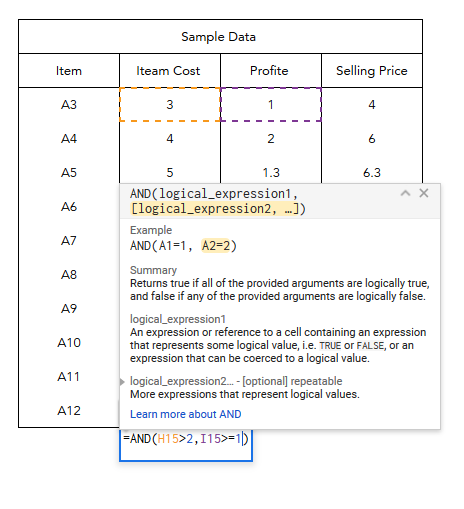1. And

=AND(A1="GOOD”, B2>10)1. Proper

=PROPER(A1)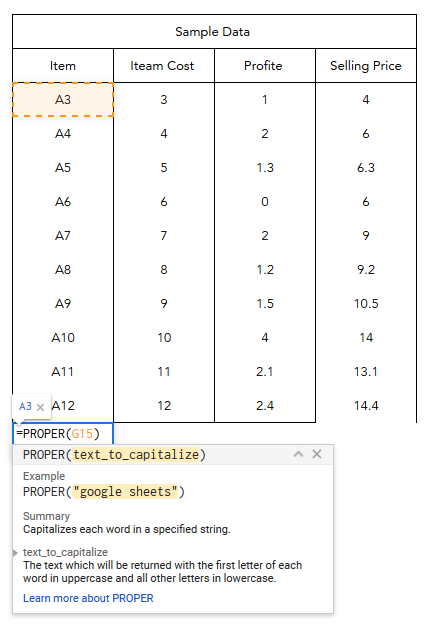1. Conditional Formatting

No Formula for this but you can select: Home –> Styles –> Conditional formatting, you can select many options that will give outputs if certain things are true. You can do a lot of this with the formulas mentioned before, but why not let Excel do the hard work.

1. Index + match

=INDEX(list of values, MATCH(what you want to lookup, lookup column, sorting identifier))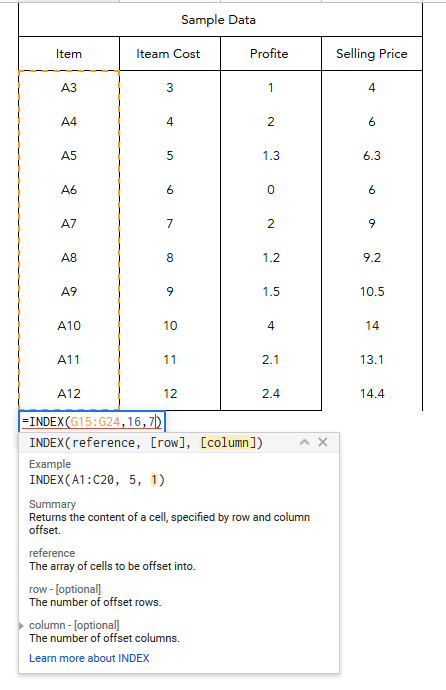#Tips#Formulas

Create Awesome Flowcharts In Excel

Some point in the past was the way to reference the last value on a row or on a column